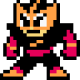## Week 7: Scribby’s First Steps

This week, I worked solely on the Prolog natural language parsing component of the project.  This is basically a set of grammar rules along with very simple code-generation based on the results of the parse. I made quite a bit of progress this week… Basic movement commands are now parsed, along with quantities and conversions of a handful of different units. For example, you can now tell the Scribbler such things as:

* go forward for 3 seconds and then turn left
* drive forward three feet and then turn right 180 degrees
* move backwards 6.5 inches

And so on and so forth. Here is a sample run:

Enter a command: go forward two feet, turn right 90 degrees, drive backwards for three seconds
Prolog query executed: parseToCode([go,forward,two,feet,and,turn,right,90,degrees,and,drive,backwards,for,three,seconds], Status, CodeOut).
Status: valid
CodeOut: [‘forward(1.0, 4.1)’, ‘turnRight(1.0, 0.7875)’, ‘backward(1.0, 3)’]

A big component that I had to work on this week was english -> integer translation. It actually requires quite a bit of code to convert an English phrase like “nine hundred forty two point threee” to “942.3”!

My next task is to add some “fun” commands to the program; beeping, taking pictures, and maybe a wander/doodle capability.  I would also like to add some flavorful error messages to return upon a failed parse.

Another potential goal is to add conditional statements to the parser, i.e. parsing of a statement like “go forward until you hit a wall and then turn left and take a picture.”

Here is the source code for the Prolog parser as it stands today…

```% Natural Language Parser for Scribbler II Robot
% by Justin Mangue, 2013
%
% References:
% * "The Art of Prolog", Sterling & Shapiro, 1986.

parseToCode(Sentence, Status, CodeListOut) :-
compound_sentence(ParseList, Sentence, []),
Status = valid,
generate_code_list(ParseList, [], CodeListOut),
!.

parseToCode(Sentence, Status, CodeOut) :-
command_phrase(Parse, Sentence, []),
Status = valid,
generate_code_list([Parse], [], CodeOut),
!.

parseToCode(Sentence, Status, CodeOut) :-
+ command_phrase(_, Sentence, []),
Status = invalid_sentence,
CodeOut = 'null',
!.

generate_code_list([], CodeList, CodeList).
generate_code_list([H|T], CodeList, CodeListOut) :-
generate_code(H, CodeLineOut),
append(CodeList, [CodeLineOut], NewCodeList),
generate_code_list(T, NewCodeList, CodeListOut).

generate_code(move(forward,S), CodeOut) :-
string_to_atom(Keyword, forward),
CodeOut = [Keyword,1.0,S], !.

generate_code(move(backward,S), CodeOut) :-
string_to_atom(Keyword, backward),
CodeOut = [Keyword,1.0,S], !.

generate_code(turn(left,S), CodeOut) :-
string_to_atom(Keyword, turnLeft),
CodeOut = [Keyword,1.0,S], !.

generate_code(turn(right,S), CodeOut) :-
string_to_atom(Keyword, turnRight),
CodeOut = [Keyword,1.0,S], !.

/*
generate_code(pic(Mode), CodeOut) :-
string_to_atom(Keyword, takePicture),
CodeOut = [[Keyword, Mode],, */

compound_sentence(Compound) -->
command_phrase(Command),
connective(and),
compound_sentence(Sentence),
{ append([Command], [Sentence], Compound_NotFlat) },
{ flatten2(Compound_NotFlat, Compound) }.

compound_sentence(Simple) --> command_phrase(Simple).

command_phrase(pic("color")) -->
[take],
[a],
[picture].

command_phrase(move(D,S)) -->
action(go),
direction(D),
[for],
unit_to_seconds(S).

command_phrase(move(D,S)) -->
action(go),
direction(D),
unit_to_seconds(S).

command_phrase(move(D,1.5)) -->
action(go),
direction(D).

command_phrase(turn(D,S)) -->
action(turn),
direction(D),
unit_to_seconds(S).

command_phrase(turn(D,S)) -->
action(turn),
direction(D),
{ S is 90*(3.15 / 360) }.  % 90 degrees by default

unit_to_seconds(S) -->
to_number(S), { + S = 1 }, [seconds] ;
to_number(S), { S = 1 }, [second].

unit_to_seconds(S) -->
to_number(Feet), { + Feet = 1 }, { S is Feet * 2.05 }, [feet] ;
to_number(Feet), { Feet = 1 }, { S is 2.05 }, [foot].

unit_to_seconds(S) -->
to_number(Inches), { + Inches = 1 }, { S is Inches * (2.05 / 12) }, [inches] ;
to_number(Inches), { Inches = 1 }, { S is (2.05 / 12) }, [inch].

unit_to_seconds(S) -->
to_number(Degrees), { + Degrees = 1 }, { S is Degrees * (3.15 / 360) }, [degrees] ;
to_number(Degrees), { Degrees = 1 }, { S is (3.15 / 360) }, [degree].

action(go) --> [go].
action(go) --> [move].
action(go) --> [drive].
action(go) --> [roll].

action(turn) --> [turn].
action(turn) --> [rotate].

action(spin) --> [spin].

connective(and) --> [and], [then].
connective(and) --> [and].
connective(and) --> [then].

direction(forward) --> [forward].
direction(forward) --> [forwards].
direction(backward) --> [backward].
direction(backward) --> [backwards].
direction(left) --> [left].
direction(left) --> [counter-clockwise].
direction(right) --> [right].
direction(right) --> [clockwise].

% English number recognition from 0.0 - 9999.9

to_number(N) --> num(N1), [point], digit(N2), { N is N1 + (0.1 * N2) }.
to_number(N) --> num(N).

num(0) --> [zero].
num(N) --> xxxx(N).
num(N) --> xxx(N).
num(N) --> xx(N).
num(N) --> digit(N).

num(N) --> [N], { number(N) }.

xxxx(N) --> digit(D), [thousand], xxx(N1), { N is D*1000+N1 }.
xxx(N) --> digit(D), [hundred], rest_xxx(N1), { N is D*100+N1 }.

rest_xxx(0) --> [].
rest_xxx(N) --> [and], xx(N).
rest_xxx(N) --> xx(N).

xx(N) --> digit(N).
xx(N) --> teen(N).
xx(N) --> tens(T), rest_xx(N1), { N is T+N1 }.

rest_xx(0) --> [].
rest_xx(N) --> digit(N).

digit(1) --> [one].
digit(2) --> [two].
digit(3) --> [three].
digit(4) --> [four].
digit(5) --> [five].
digit(6) --> [six].
digit(7) --> [seven].
digit(8) --> [eight].
digit(9) --> [nine].

teen(10) --> [ten].
teen(11) --> [eleven].
teen(12) --> [twelve].
teen(13) --> [thirteen].
teen(14) --> [fourteen].
teen(15) --> [fifteen].
teen(16) --> [sixteen].
teen(17) --> [seventeen].
teen(18) --> [eighteen].
teen(19) --> [nineteen].

tens(20) --> [twenty].
tens(30) --> [thirty].
tens(40) --> [forty].
tens(50) --> [fifty].
tens(60) --> [sixty].
tens(70) --> [seventy].
tens(80) --> [eighty].
tens(90) --> [ninety].

sublist(S, L) :-
append(_, L2, L),
append(S, _, L2).

flatten2([], []) :- !.
flatten2([L|Ls], FlatL) :-
!,
flatten2(L, NewL),
flatten2(Ls, NewLs),
append(NewL, NewLs, FlatL).
flatten2(L, [L]).
```